UMLS. CSP-HL7-ICD9CM-NCI-NDFRT-RXNORM

# Equation

[A statement written in symbols that two objects, e.g. mathematical expressions, or arrays of symbols representing a chemical reaction, are equal under certain conditions. Equations are usually and sometimes tacitly accompanied by the requirement to establish these conditions. In the case where mathematical or chemical objects contain arbitrary variables, such a requirement may mean finding those values of the variables that turn the equation into equality. Equations are sometimes called more explicitly as conditional equations. ( NCI )]
UMLS (NCI) C0552449

Conceptual Entity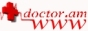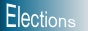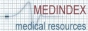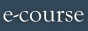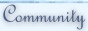home Dictionaries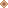Armenian-EnglishEnglish-ArmenianMeSHFeedbacktop